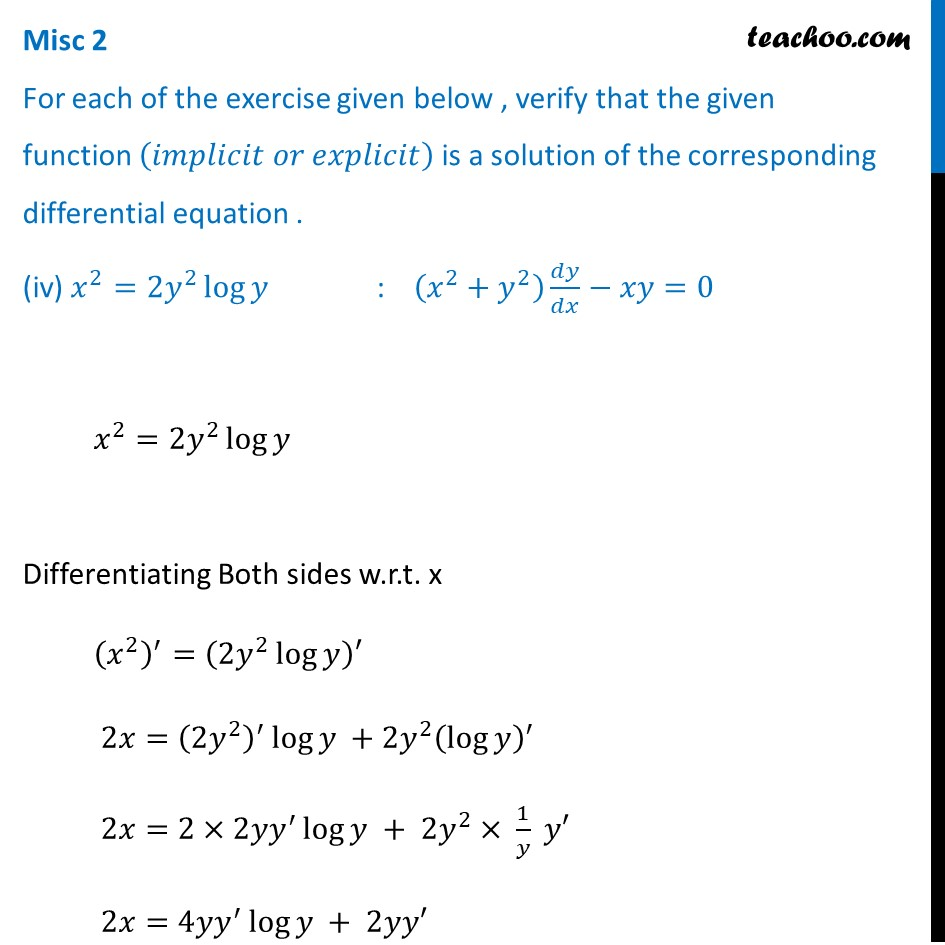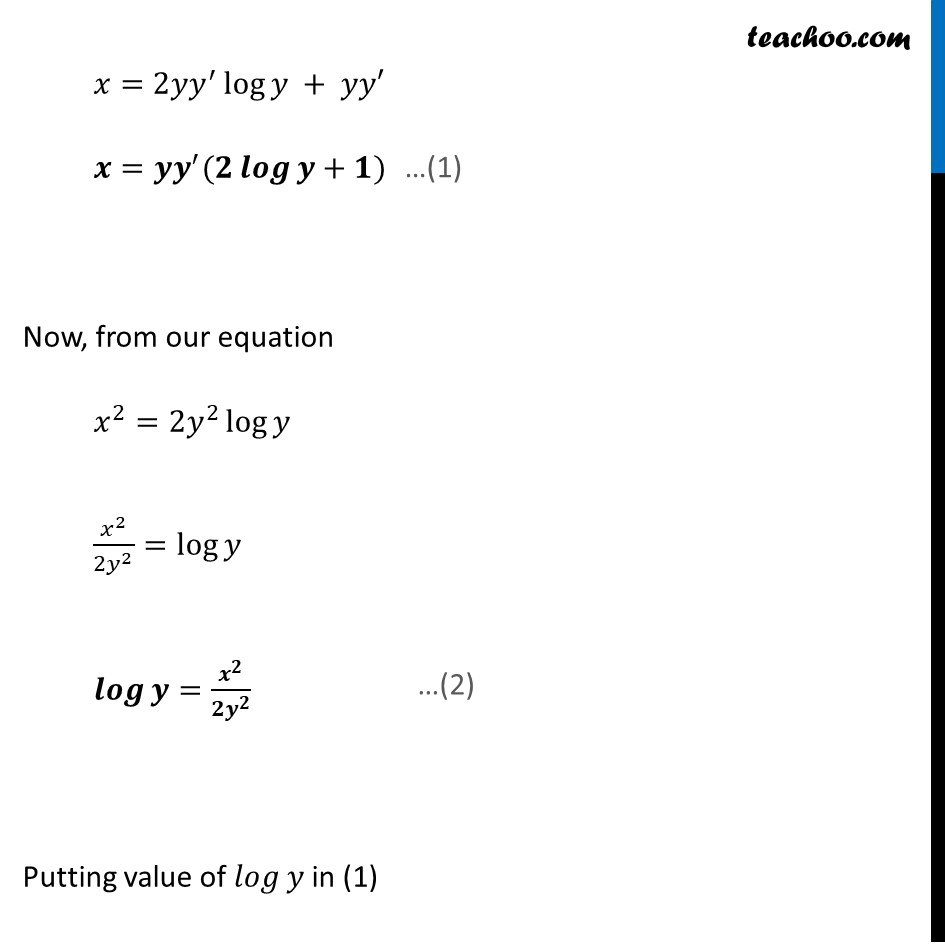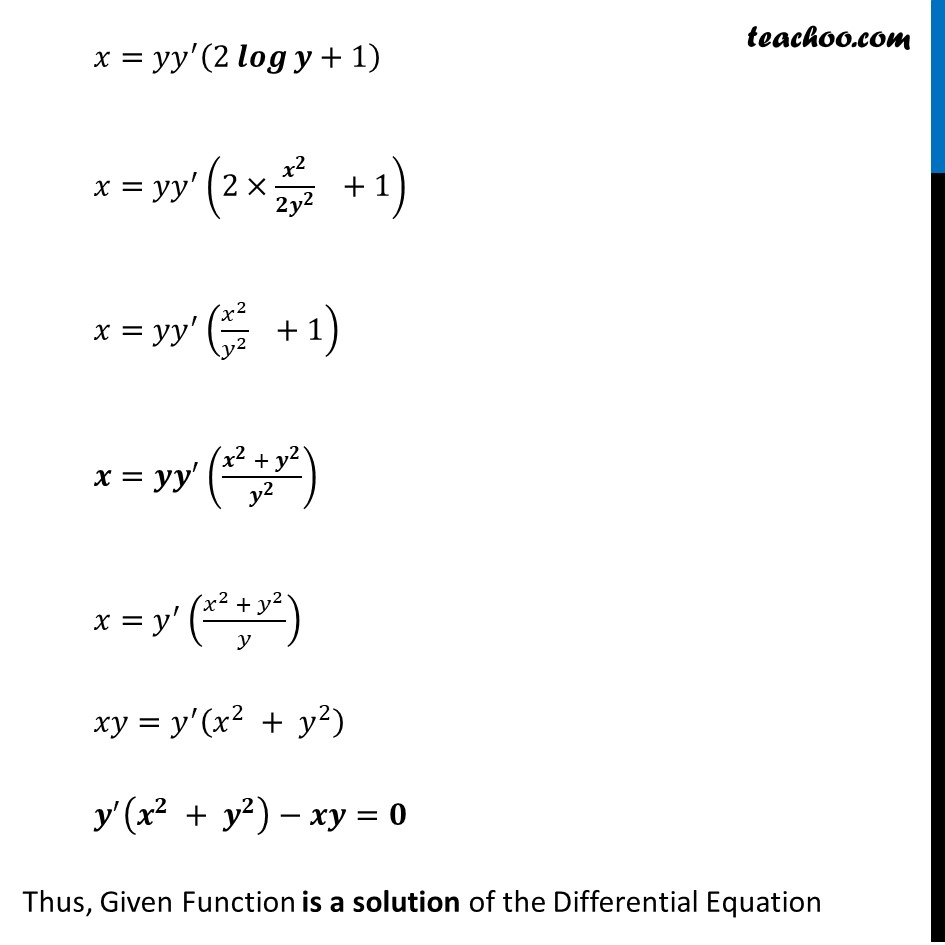Miscellaneous

Chapter 9 Class 12 Differential Equations
Serial order wiseLearn in your speed, with individual attention - Teachoo Maths 1-on-1 Class

### Transcript

Misc 2 For each of the exercise given below , verify that the given function (𝑖𝑚𝑝𝑙𝑖𝑐𝑖𝑡 𝑜𝑟 𝑒𝑥𝑝𝑙𝑖𝑐𝑖𝑡) is a solution of the corresponding differential equation . (iv) 𝑥^2=2𝑦^2 log⁡𝑦 : (𝑥^2+𝑦^2 ) 𝑑𝑦/𝑑𝑥−𝑥𝑦=0 𝑥^2=2𝑦^2 log⁡𝑦 Differentiating Both sides w.r.t. x (𝑥^2 )^′=(2𝑦^2 log⁡𝑦 )′ 2𝑥=(2𝑦^2 )^′ log⁡𝑦 +2𝑦^2 (log⁡𝑦 )^′ 2𝑥=2×2𝑦𝑦^′ log⁡𝑦 + 2𝑦^2× 1/𝑦 𝑦′ 2𝑥=4𝑦𝑦^′ log⁡𝑦 + 2𝑦𝑦′ 𝑥=2𝑦𝑦^′ log⁡𝑦 + 𝑦𝑦′ 𝒙=〖𝒚𝒚〗^′ (𝟐 𝒍𝒐𝒈⁡𝒚+𝟏) Now, from our equation 𝑥^2=2𝑦^2 log⁡𝑦 𝑥^2/(2𝑦^2 )=log⁡𝑦 𝒍𝒐𝒈⁡𝒚=𝒙^𝟐/(𝟐𝒚^𝟐 ) Putting value of 𝑙𝑜𝑔⁡𝑦 in (1) 𝑥=〖𝑦𝑦〗^′ (2 𝒍𝒐𝒈⁡𝒚+1) 𝑥=〖𝑦𝑦〗^′ (2×𝒙^𝟐/(𝟐𝒚^𝟐 ) " " +1) 𝑥=〖𝑦𝑦〗^′ (𝑥^2/𝑦^2 " " +1) 𝒙=〖𝒚𝒚〗^′ ((𝒙^𝟐 + 𝒚^𝟐)/𝒚^𝟐 ) 𝑥=𝑦^′ ((𝑥^2 + 𝑦^2)/𝑦) 𝑥𝑦=𝑦^′ (𝑥^2 + 𝑦^2 ) 𝒚^′ (𝒙^𝟐 + 𝒚^𝟐 )−𝒙𝒚=𝟎 Thus, Given Function is a solution of the Differential Equation